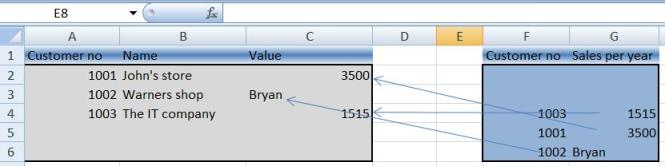Welcome to officecoding...

# What function should I use to locate and retrieve data or its associated data?

### What function should I use to locate and retrieve data or its associated data?

April 18, 2017
|
|

VLOOKUP and HLOOKUP are the functions that can do this. VLOOKUP is the function that will search data vertically while HLOOKUP is the function that will search data horizontally.

The below image shows how VLOOKUP Function works:In the above example,  Columns A to C is the first table or dataset.    Columns F to G is the second table or dataset.  To use VLOOKUP, the two (2) tables should have a common data.   The common data is the connection of the two different tables which is needed to retrieve the value from one table and place it in another table.

In the above example, the common data is the Customer No.  This is the value to use to retrieve Sales per year which is in Column G and place it in Column C.  The below formula should be entered in the cell with address C2 and copied down to the last cell of the first table which in this case is C4:

=VLOOKUP(A2,\$F\$2:\$G\$6,2,FALSE)

where the above formula by description is:

=VLOOKUP(Value to locate or look, Table to search, Column number of the data to retrieve in the table to search, Exact Match [False] or Approximate Value [True])

1. A2 contains the value to locate in the second table
2. \$F\$2:\$G\$6 is the address of the table to search and where the value to retrieve can be found.  The dollar sign (\$) makes the address fixed.  When the formula is copied down to the last cell of the first table, this address will not change.
3. 2 is the column number in the table to search where the value to retrieve is located.  Customer No. is Column F which is column number 1 and Sales Per Year is Column G which is column number 2 in the table to search.
4. The False command in the formula is to find the exact match of the Customer No. in Column A in the Customer No. in Column F